# Discussion about train loss and valid loss

Hi I am a beginner in deep learning and pytorch. Would like to clarify the following outcomes I got.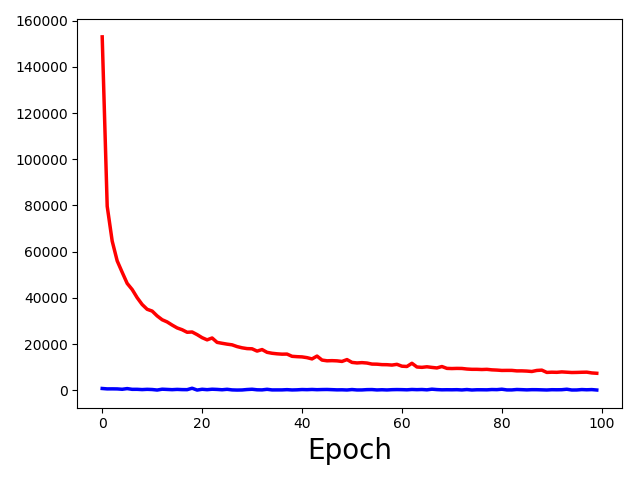This graph contains both train loss and valid loss. The train is red and valid is blue. My question is:

1. What can I learn from this graph about the model I am working with.This is just the valid loss from the upper one.
2. What can I infer from this one?

Thanks

Are you summing the loss or is it the mean value?
Which loss are you using and what target (i.e. how many classes, if classification use case)?

This is for training…

`````` for epoch in range(num_epochs):
running_loss = 0
for i,sample in enumerate(train_loader):
.
.
.
output = net(mr)
optimizer.zero_grad()
loss = criterion(ct,output)
loss.backward()
optimizer.step()
running_loss += loss.item()
mean_loss = running_loss/(i+1)

``````

and this is for validation:

``````        for i, sample in enumerate(valid_loader):
running_loss = 0
with torch.no_grad():
mr = sample.float().to(device)
ct = sample.float().to(device)
output = net(mr)
loss = criterion(ct, output)
running_loss += loss.item()
mean_loss = running_loss / (i + 1)
print(
'epoch: ...
``````

1. from the code I am using above I am summing the loss per epoch in `running_loss` and the dividing it to get `mean_loss` per epoch.
2. I am using `criterion = nn.MSELoss()` loss.
3. input size is `5,1,32,32,32` and trying to synthesize output.

Thanks for the information.
What is the range of your target?

You mean data values?
Target voxel intensities range from -1010 to 200/300

Does the train and validation set contain the same intensity values?
Also, do the training inputs contain a lot of air (-1000HU), while the validation data contain more organs?

Based on the loss, it seems your model might start with E(output)=0, while the target is a lot of -1000s.

Normalizing the output to e.g. `[-1, 1]` might help.
For the validation/test you could unnormalize the targets again and compute the real MSE.

Does the train and validation set contain the same intensity values?
Out of 20 3D subject images(172x220x156), I have split the train-valid-test as 16-2-2. So the train and validation sets are not from the same subject.

Also, do the training inputs contain a lot of air (-1000HU), while the validation data contain more organs?
The train and validation patches are taken in a similar pattern, so it should not be dissimilar drastically.

Could you be a little specific about the normalization?
Shall I normalize both input and output from `[-1,1]` and train the network?

I would start by standardizing the input (zero mean, unit variance) similar to the preprocessing pipeline for images.
Regarding the target, I would also start by normalizing it to e.g. `[0, 1]` and check both outputs.

Could you run a small test by printing the mean value of the first input training sample and the first validation sample as well as the mean values of the outputs of your model for these targets?
The discrepancy between these losses is so huge, that I would assume a total domain mismatch.

from the code below:

``````    for epoch in range(max_epochs):
.
.
for i,sample in enumerate(train_loader):
.
.
mr = sample.float().to(device)   #torch.Size([batch_size, 1, 32, 32, 32])
print(mr.mean())
ct = sample.float().to(device)
print(ct.mean())
``````

and the mean of the train and validation samples are.

``````train directory has 8000 samples
valid directory has 1000 samples
training ...
train input: 46.612483978271484
train target: 120.2796859741211
train output: 0.11282896995544434

validation input: 46.15531921386719
validation target: -71.48211669921875
validation output: -0.17590099573135376
``````

Do you have 20 samples in total and split them in 16-2-2?
Since your targets seem to have a high variance I’m not sure, if your model can learn anything useful from the data.

You could try to normalize it, as described, and hope for the best.

1 Like

I worked with the same images in 2D network. I didnt normalize or standardize the data but it worked pretty well. I had used metrics like MAE,MSE and PSNR those were in workable range.
The 2D architecture I had used were UNet and GAN with GDL loss.
So I wanted to transfer the same pipeline to 3D network.
I will try the normalizing and see how it works.

I was wondering why for the same code and data validation losses are so smaller than training losses??
Any idea?

It’s a bit hard to tell, but you might just be unlucky in that your validation data values are closer to the current output (`0`) as indicated my the mean values.

Hey,
I took `mean` and `std` of the train and validation dataset and standardized both input and target to zero mean, unit variance.

``````MR = (MR - meanMR) / stdMR
``````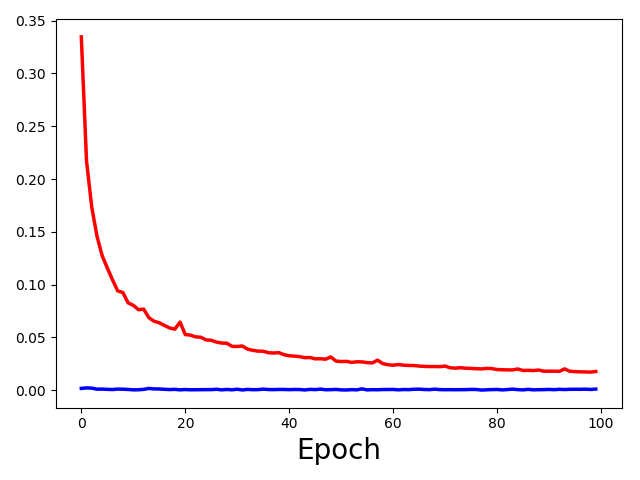Train(red) and validation(blue) loss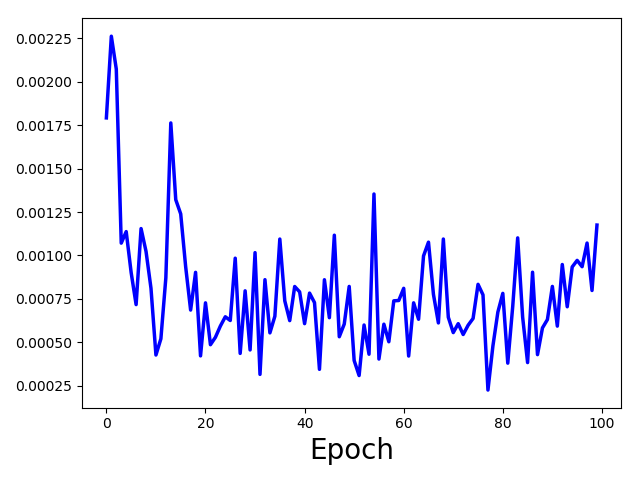After that I tried with normalization [0,1] the ground truth and input train and validation set.
The results are kinda similar.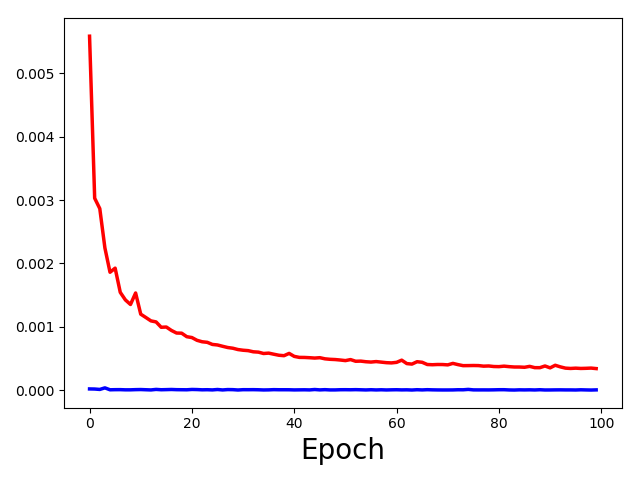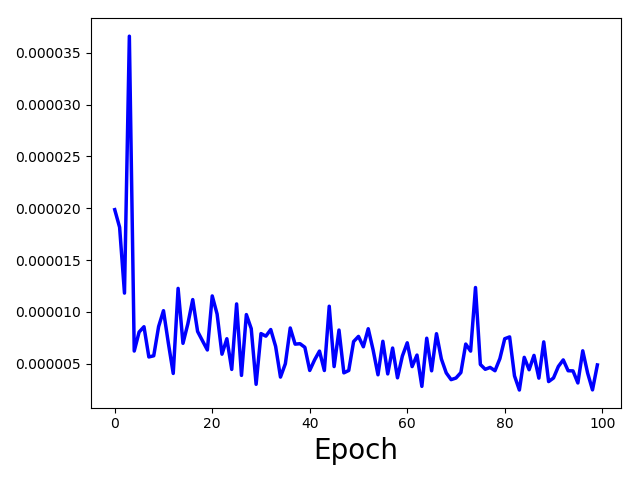any thoughts?

Could you print histograms for the pixel/voxel values of your training and validation images?

Hi @ptrblck,
I did some debugging in the validation codes and corrected the `running_loss` line which should start before validation epoch iteration.
Now the loss looks like the following based on 100 patches/image. (1600 train and 200 valid)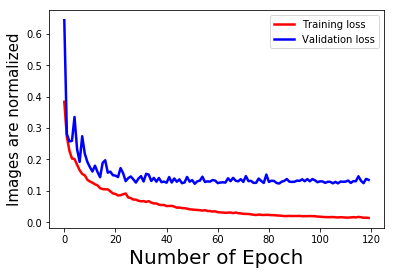What do you think about it?

Is it overfitting as the training loss is lower than the validation loss? But how to define lower?

Good to hear you’ve found a bug!

The loss curves now look like usual loss curves.Yes, your model is overfitting, as the training loss decreases while the validation loss hits a plateau.
If you continue training, the validation loss will probably even increase again.

You could counter it with adding some regularization or reduce the model capacity (e.g. using dropout, fewer parameters etc.).

Right now I am using minimum validation loss as my chosen criteria to save the model.
What do you think about it?
Do you think training the model with more number of patches gonna change the loss graph above like reducing the valid loss or so?

That makes sense, as it’s basically using early stopping.

I’m really not sure, as I don’t know how the number of patches affects the capacity of the model.
Try to change it and observe if the gap between the loss curves closes or spreads.